# T.INV.2T Function

Calculates the two-tailed Student's T-Distribution

## What is the T.INV.2T Function?

The T.INV.2T Function is categorized under Excel Statistical functions. It will calculate the two-tailed Student’s T-Distribution. The Student’s T-Distribution is a continuous probability distribution that is frequently used for testing hypotheses on small sample data sets.

In financial analysis, T.INV.2T is often used in examining the risk-return relationship of a portfolio. The T.INV.2T function is the updated version of the TINV function.

### Formula

=T.INV.2T(probability,deg_freedom)

The T.INV.2T function uses the following arguments:

1. Probability (required argument) – This is the probability associated with the Student’s T Distribution. It should be between 0 and 1.
2. Deg_freedom (required argument) – The number of degrees of freedom with which to characterize the distribution.

### How to use the T.INV.2T Function in Excel?

To understand the uses of the T.INV.2T function, let’s consider a few examples:

#### Example 1

Suppose we are given the following data: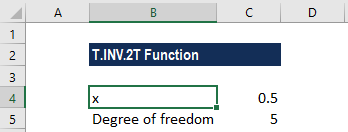If we want to calculate the value of the function for a probability of 0.5, the formula to use will be: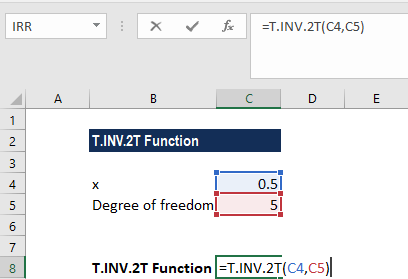We get the result below: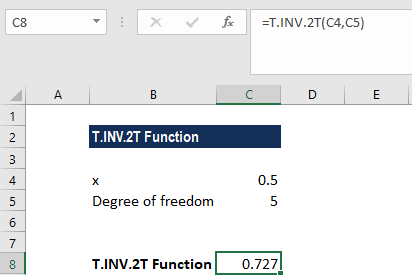So, the T-value of the Student’s T-distribution for the data above is 0.727.

#### Example 2

We wish to calculate a one-tailed t-value that will be returned by replacing probability with 2*probability. Suppose we are given the following data: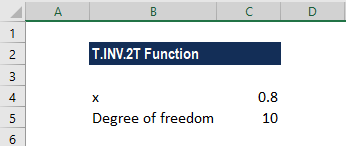For a probability of 0.8 and a degree of freedom of 10, the two-tailed value is calculated using the formula below: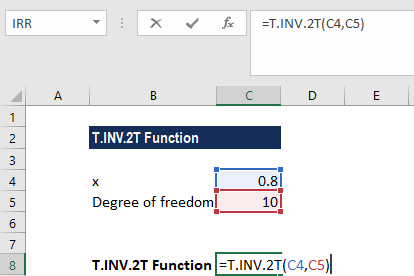We get the result below: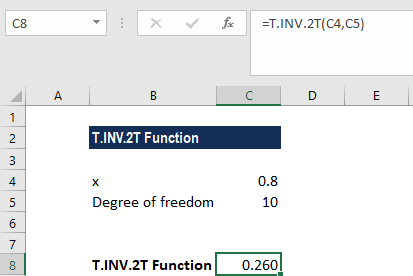The above value is a two-tailed value. The one-tailed value for the same probability and degrees of freedom can be calculated as shown below: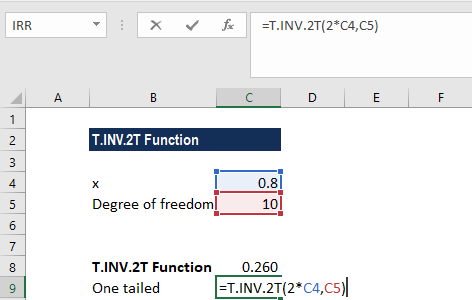We get the following result: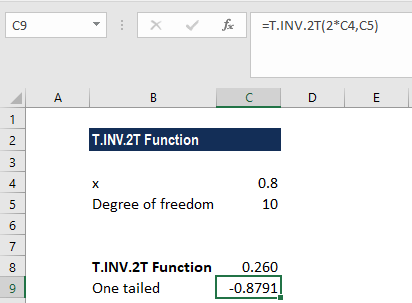### Things to remember about the T.INV.2T Function

1. #NUM! error – Occurs when:
• The given probability is less than zero or the probability is equal to zero or is greater than one.
• The given degree of freedom is less than 1.
2. #VALUE! error – Occurs when one or both of the supplied arguments are non-numeric.
3. If the deg_freedom argument is not an integer, it is truncated.

Thanks for reading CFI’s guide to the Excel T.INV.2T function. By taking the time to learn and master Excel functions, you’ll significantly speed up your financial analysis. To learn more, check out these additional CFI resources:

• Excel Functions for Finance